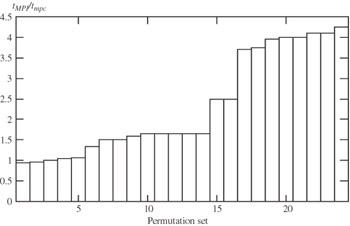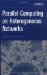# 9.3. NUMERICAL INTEGRATION

## 9.2. N-BODY PROBLEM

In this section we look at some experimental results of the mpC N-body application presented in Section 7.1. The run time of the mpC program was compared to a carefully written MPI counterpart. Three workstations—SPARCstation 5 (hostname gamma), SPARCclassic (omega), and SPARCstation 20 (alpha), connected via 10Mbits Ethernet—were used as the NoC. There were 23 other computers in the same segment of the local network. LAM MPI version 5.2  was used as the communication platform.

The computing space of the mpC programming environment consisted of 15 processes, 5 processes running on each workstation. The dispatcher ran on gamma and used the following relative performances of the workstations obtained automatically upon the creation of the virtual parallel machine: 1150 (gamma), 331 (omega), and 1662 (alpha).

The MPI program was written in a way that would minimize communication overhead. All our experiments dealt with nine groups of bodies. Three MPI processes were mapped to gamma, 1 process to omega, and 5 processes to alpha, providing the optimal mapping if the numbers of bodies in these groups were equal to each other.

The first experiment compared the mpC and MPI programs for homogeneous input data where all groups consisted of the same number of bodies. In effect, it showed how much time is lost in using mpC instead of pure MPI. It turned out that the run time of the MPI program consisted of about 95% to 97% of the run time of the mpC program. The loss in performance was 3% to 5%.

The second experiment compared these programs for heterogeneous input data. The groups consisted of 10, 10, 10, 100, 100, 100, 600, 600, and 600 bodies correspondingly.

The run time of the mpC program did not depend on the order of the numbers. The dispatcher selected three different groups of numbers:

• Four processes on gamma for virtual processors of network g computing two 10-body groups, one 100-body group, and one 600-body group.

• Three processes on omega for virtual processors computing one 10-body group and two 100-body groups.

• Two processes on alpha for virtual processors computing two 600-body groups.

The mpC program took 94 seconds to simulate 15 hours of the galaxy evolution.

The run time of the MPI program essentially depended on the order of the selected numbers. It took from 88 to 391 seconds to simulate 15 hours of the galaxy evolution dependent on the particular order. Figure 9.13 shows the relative runtime of the MPI and mpC programs for different permutations of these numbers. All possible permutations can be broken down into 24 disjoint subsets of the same power in such a way that if two permutations belong to the same subset, the corresponding run times are equal to each other. We let these subsets be numerated so that as the number of the subset has became greater, the MPI program took longer to run. In Figure 9.13 we represent each such a subset by a bar, whose height is equal to the corresponding value tMPI/tmpC. As can be seen in the figure, for almost all input data the runtime of the MPI program exceeds (and often, essentially) the run time of the mpC program.Figure 9.13: Speedups for different permutations of the numbers of bodies in groups.Parallel Computing on Heterogeneous Networks (Wiley Series on Parallel and Distributed Computing)
ISBN: B000W7ZQBO
EAN: N/A
Year: 2005
Pages: 95